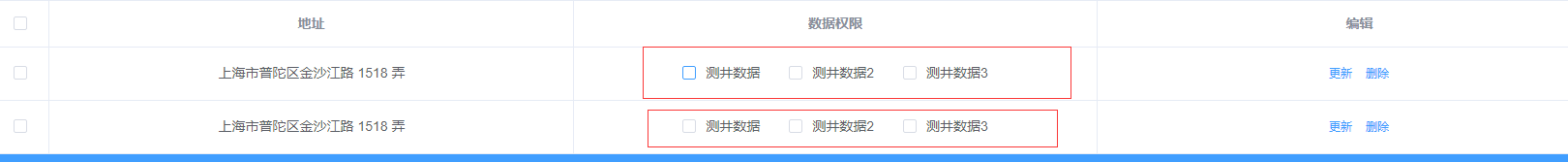vue中怎么获取Checkbox多选值5个回答2 年多之前 回复qq_41126602 <div class="e_ipt"> <div class="e_iptTle">登录密码</div> <div class="e_iptTxt"> <input type="text" class="e_input" name="cPwd" v-model="dataList.cPwd" /> </div> </div>
2 年多之前 回复qq_41126602 多组数据 你可 已设置一个 空的 集和 把你修改的值 赋给集合
2 年多之前 回复2 年多之前 回复

var selectedData = [];
\$(":checkbox:checked").each(function(){
var tablerow = \$(this).parent("tr");
var code = tablerow.find("[name='p_code']").val();
var name= tablerow.find("[name='p_name']").val();
var price= tablerow.find("[name='p_price']").val();
selectedData.push({Code:code,Name:name,Price:price});
});clken 上面几个答案都是垃圾，只有你的是对的。 v-model 绑定的值就是选中的值。
10 个月之前 回复